# 1.6 Decimal fractions

 Page 1 / 1
This module is from Elementary Algebra by Denny Burzynski and Wade Ellis, Jr. This chapter contains many examples of arithmetic techniques that are used directly or indirectly in algebra. Since the chapter is intended as a review, the problem-solving techniques are presented without being developed. Therefore, no work space is provided, nor does the chapter contain all of the pedagogical features of the text. As a review, this chapter can be assigned at the discretion of the instructor and can also be a valuable reference tool for the student.

## Overview

• Decimal Fractions
• Adding and Subtracting Decimal Fractions
• Multiplying Decimal Fractions
• Dividing Decimal Fractions
• Converting Decimal Fractions to Fractions
• Converting Fractions to Decimal Fractions

## Decimal fractions

Fractions are one way we can represent parts of whole numbers. Decimal fractions are another way of representing parts of whole numbers.

## Decimal fractions

A decimal fraction is a fraction in which the denominator is a power of 10.

A decimal fraction uses a decimal point to separate whole parts and fractional parts. Whole parts are written to the left of the decimal point and fractional parts are written to the right of the decimal point. Just as each digit in a whole number has a particular value, so do the digits in decimal positions.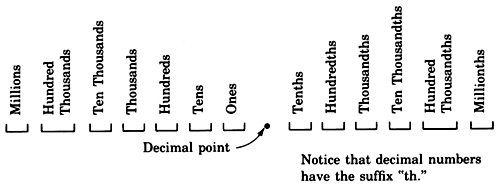## Sample set a

The following numbers are decimal fractions.

$\begin{array}{l}57.9\\ \text{The\hspace{0.17em}9\hspace{0.17em}is\hspace{0.17em}in\hspace{0.17em}the}\text{\hspace{0.17em}}tenths\text{\hspace{0.17em}}\text{position}.\text{\hspace{0.17em}}57.9=57\frac{9}{10}.\end{array}$

$\begin{array}{l}6.8014\text{\hspace{0.17em}}\\ \text{The\hspace{0.17em}8\hspace{0.17em}is\hspace{0.17em}in\hspace{0.17em}the\hspace{0.17em}}tenths\text{\hspace{0.17em}position}\text{.\hspace{0.17em}}\\ \text{The\hspace{0.17em}0\hspace{0.17em}is\hspace{0.17em}in\hspace{0.17em}the\hspace{0.17em}}hundredths\text{\hspace{0.17em}position}\text{.\hspace{0.17em}}\\ \text{The\hspace{0.17em}1\hspace{0.17em}is\hspace{0.17em}in\hspace{0.17em}the\hspace{0.17em}}thousandths\text{\hspace{0.17em}position}\text{.\hspace{0.17em}}\\ \text{The\hspace{0.17em}4\hspace{0.17em}is\hspace{0.17em}in\hspace{0.17em}the\hspace{0.17em}ten\hspace{0.17em}}thousandths\text{\hspace{0.17em}position}\text{.\hspace{0.17em}}\\ 6.8014=6\frac{8014}{10000}.\end{array}$

## Adding and subtracting decimal fractions

To add or subtract decimal fractions,
1. Align the numbers vertically so that the decimal points line up under each other and corresponding decimal positions are in the same column. Add zeros if necessary.
2. Add or subtract the numbers as if they were whole numbers.
3. Place a decimal point in the resulting sum or difference directly under the other decimal points.

## Sample set b

Find each sum or difference.

\begin{array}{l}9.183+2.140\\ \begin{array}{rrr}\text{\hspace{0.17em}}↓\hfill & \hfill & \text{The\hspace{0.17em}decimal\hspace{0.17em}points\hspace{0.17em}are\hspace{0.17em}aligned\hspace{0.17em}in\hspace{0.17em}the\hspace{0.17em}same\hspace{0.17em}column}\text{.\hspace{0.17em}}\hfill \\ \hfill \text{9}\text{.183}& \hfill & \hfill \\ \hfill \text{+}\underset{¯}{\text{\hspace{0.17em}2}\text{.140}}& \hfill & \hfill \\ \hfill \text{11}\text{.323}& \hfill & \hfill \end{array}\end{array}

\begin{array}{l}841.0056\text{\hspace{0.17em}}+\text{\hspace{0.17em}}47.016\text{\hspace{0.17em}}+\text{\hspace{0.17em}}19.058\text{\hspace{0.17em}}\\ \begin{array}{rrr}\hfill ↓& \hfill & \text{The\hspace{0.17em}decimal\hspace{0.17em}points\hspace{0.17em}are\hspace{0.17em}aligned\hspace{0.17em}in\hspace{0.17em}the\hspace{0.17em}same\hspace{0.17em}column}\text{.\hspace{0.17em}}\hfill \\ \hfill 841.0056& \hfill & \hfill \\ \hfill 47.016& \hfill & \text{Place\hspace{0.17em}a\hspace{0.17em}0\hspace{0.17em}into\hspace{0.17em}the\hspace{0.17em}thousandths\hspace{0.17em}position}\text{.}\hfill \\ \hfill +\text{\hspace{0.17em}}\underset{¯}{19.058}& \hfill & \text{Place\hspace{0.17em}a\hspace{0.17em}0\hspace{0.17em}into\hspace{0.17em}the\hspace{0.17em}thousandths\hspace{0.17em}position}\text{.\hspace{0.17em}}\hfill \\ \hfill ↓& \hfill & \text{The\hspace{0.17em}decimal\hspace{0.17em}points\hspace{0.17em}are\hspace{0.17em}aligned\hspace{0.17em}in\hspace{0.17em}the\hspace{0.17em}same\hspace{0.17em}column}\text{.\hspace{0.17em}}\hfill \\ \hfill 841.0056& \hfill & \hfill \\ \hfill 47.0160& \hfill & \hfill \\ \hfill +\text{\hspace{0.17em}}\underset{¯}{19.0580}& \hfill & \hfill \\ \hfill 907.0796& \hfill & \hfill \end{array}\end{array}

\begin{array}{l}16.01\text{\hspace{0.17em}}-\text{\hspace{0.17em}}7.053\\ \begin{array}{rrr}\hfill ↓& \hfill & \text{The\hspace{0.17em}decimal\hspace{0.17em}points\hspace{0.17em}are\hspace{0.17em}aligned\hspace{0.17em}in\hspace{0.17em}the\hspace{0.17em}same\hspace{0.17em}column}\text{.\hspace{0.17em}}\hfill \\ \hfill 16.01& \hfill & \text{Place\hspace{0.17em}a\hspace{0.17em}0\hspace{0.17em}into\hspace{0.17em}the\hspace{0.17em}thousandths\hspace{0.17em}position}\text{.\hspace{0.17em}}\hfill \\ \hfill -\text{\hspace{0.17em}}\underset{¯}{7.053}& \hfill & \hfill \\ \hfill ↓& \hfill & \text{The\hspace{0.17em}decimal\hspace{0.17em}points\hspace{0.17em}are\hspace{0.17em}aligned\hspace{0.17em}in\hspace{0.17em}the\hspace{0.17em}same\hspace{0.17em}column}\text{.\hspace{0.17em}}\hfill \\ \hfill 16.010& \hfill & \hfill \\ \hfill -\text{\hspace{0.17em}}\underset{¯}{7.053}& \hfill & \hfill \\ \hfill 8.957& \hfill & \hfill \end{array}\end{array}

## Multiplying decimal fractions

To multiply decimals,
1. Multiply tbe numbers as if they were whole numbers.
2. Find the sum of the number of decimal places in the factors.
3. The number of decimal places in the product is the sum found in step 2.

## Sample set c

Find the following products.

$6.5×4.3$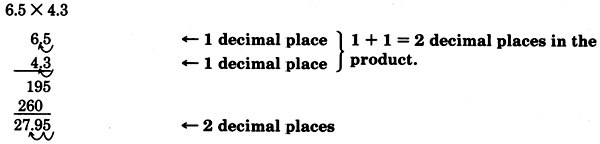$6.5×4.3=27.95$

$23.4×1.96$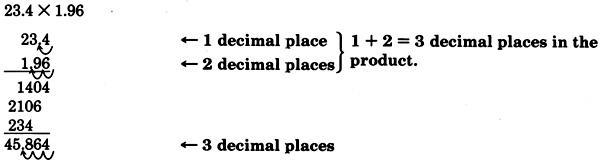$23.4×1.96=45.864$

## Dividing decimal fractions

To divide a decimal by a nonzero decimal,
1. Convert the divisor to a whole number by moving the decimal point to the position immediately to the right of the divisor’s last digit.
2. Move the decimal point of the dividend to the right the same number of digits it was moved in the divisor.
3. Set the decimal point in the quotient by placing a decimal point directly above the decimal point in the dividend.
4. Divide as usual.

## Sample set d

Find the following quotients.

$32.66÷7.1$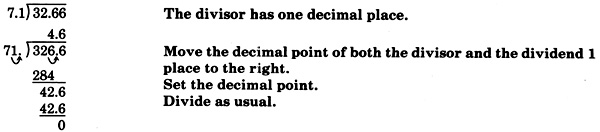$\begin{array}{l}32.66÷7.1=4.6\\ \begin{array}{lll}Check:\hfill & \hfill & 32.66÷7.1=4.6\text{\hspace{0.17em}}\text{if}\text{\hspace{0.17em}}4.6×7.1=32.66\hfill \\ \hfill \text{\hspace{0.17em}}4.6& \hfill & \hfill \\ \hfill \underset{¯}{\text{\hspace{0.17em}}7.1}& \hfill & \hfill \\ \hfill \text{\hspace{0.17em}}4.6& \hfill & \hfill \\ \underset{¯}{322\text{\hspace{0.17em}}\text{\hspace{0.17em}}}\hfill & \hfill & \hfill \\ 32.66\hfill & \hfill & \text{True}\hfill \end{array}\end{array}$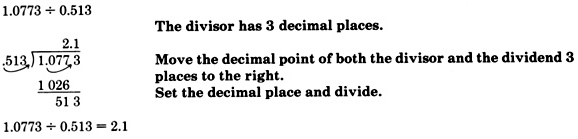Check by multiplying $2.1$ and $0.513.$ This will show that we have obtained the correct result.

$12÷0.00032$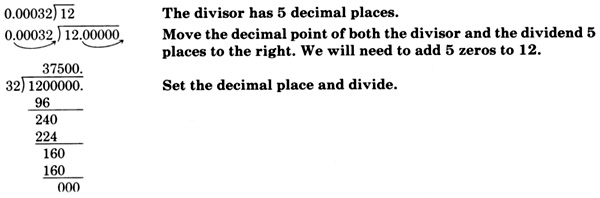## Converting decimal fractions to fractions

We can convert a decimal fraction to a fraction by reading it and then writing the phrase we have just read. As we read the decimal fraction, we note the place value farthest to the right. We may have to reduce the fraction.

## Sample set e

Convert each decimal fraction to a fraction.

$\begin{array}{l}0.6\\ 0.\underset{¯}{6}\to \text{tenths\hspace{0.17em}position}\\ \begin{array}{lll}\text{Reading:}\hfill & \hfill & \text{six\hspace{0.17em}tenths}\to \frac{6}{10}\hfill \\ \text{Reduce:}\hfill & \hfill & 0.6=\frac{6}{10}=\frac{3}{5}\hfill \end{array}\end{array}$

$\begin{array}{l}21.903\\ 21.90\underset{¯}{3}\to \text{thousandths\hspace{0.17em}position}\\ \begin{array}{ccc}\text{Reading:}& & \text{twenty-one\hspace{0.17em}and\hspace{0.17em}nine\hspace{0.17em}hundred\hspace{0.17em}three\hspace{0.17em}thousandths}\to 21\frac{903}{1000}\end{array}\end{array}$

## Sample set f

Convert the following fractions to decimals. If the division is nonterminating, round to 2 decimal places.

$\frac{3}{4}$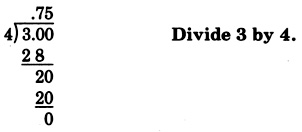$\frac{3}{4}=0.75$

$\frac{1}{5}$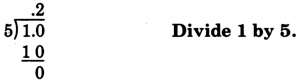$\frac{1}{5}=0.2$

$\frac{5}{6}$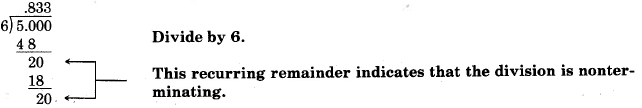$\begin{array}{llll}\frac{5}{6}\hfill & =\hfill & 0.833...\hfill & \begin{array}{l}\\ \text{We\hspace{0.17em}are\hspace{0.17em}to\hspace{0.17em}round\hspace{0.17em}to\hspace{0.17em}2\hspace{0.17em}decimal\hspace{0.17em}places}.\text{\hspace{0.17em}}\end{array}\hfill \\ \frac{5}{6}\hfill & =\hfill & 0.83\text{\hspace{0.17em}to\hspace{0.17em}2\hspace{0.17em}decimal\hspace{0.17em}places}\text{.}\hfill & \hfill \end{array}$

$\begin{array}{l}5\frac{1}{8}\\ \text{Note\hspace{0.17em}that\hspace{0.17em}}5\frac{1}{8}=5+\frac{1}{8}.\end{array}$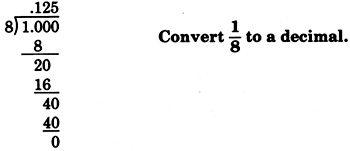$\begin{array}{l}\frac{1}{8}=.125\\ \text{Thus,\hspace{0.17em}}5\frac{1}{8}=5+\frac{1}{8}=5+.125=5.125.\end{array}$

$0.16\frac{1}{4}$

This is a complex decimal. The “6” is in the hundredths position. The number $0.16\frac{1}{4}$ is read as “sixteen and one-fourth hundredths.”

$\begin{array}{lll}0.16\frac{1}{4}=\frac{16\frac{1}{4}}{100}=\frac{\frac{16·4+1}{4}}{100}\hfill & =\hfill & \frac{\frac{65}{4}}{\frac{100}{1}}\hfill \\ \hfill & =\hfill & \frac{\stackrel{13}{\overline{)65}}}{4}·\frac{1}{\underset{20}{\overline{)100}}}=\frac{13×1}{4×20}=\frac{13}{80}\hfill \end{array}$

Now, convert $\frac{13}{80}$ to a decimal.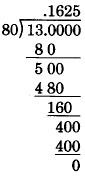$0.16\frac{1}{4}=0.1625.$

## Exercises

For the following problems, perform each indicated operation.

$1.84+7.11$

$8.95$

$15.015-6.527$

$4.904-2.67$

$2.234$

$156.33-24.095$

$.0012+1.53+5.1$

$6.6312$

$44.98+22.8-12.76$

$5.0004-3.00004+1.6837$

$3.68406$

$1.11+12.1212-13.131313$

$4.26\text{\hspace{0.17em}}·\text{\hspace{0.17em}}3.2$

$13.632$

$2.97\text{\hspace{0.17em}}·\text{\hspace{0.17em}}3.15$

$23.05\text{\hspace{0.17em}}·\text{\hspace{0.17em}}1.1$

$25.355$

$5.009\text{\hspace{0.17em}}·\text{\hspace{0.17em}}2.106$

$0.1\text{\hspace{0.17em}}·\text{\hspace{0.17em}}3.24$

$0.324$

$100\text{\hspace{0.17em}}·\text{\hspace{0.17em}}12.008$

$1000\text{\hspace{0.17em}}·\text{\hspace{0.17em}}12.008$

$12,008$

$10,000\text{\hspace{0.17em}}·\text{\hspace{0.17em}}12.008$

$75.642\text{\hspace{0.17em}}÷\text{\hspace{0.17em}}18.01$

$4.2$

$51.811\text{\hspace{0.17em}}÷\text{\hspace{0.17em}}1.97$

$0.0000448\text{\hspace{0.17em}}÷\text{\hspace{0.17em}}0.014$

$0.0032$

$0.129516\text{\hspace{0.17em}}÷\text{\hspace{0.17em}}1004$

For the following problems, convert each decimal fraction to a fraction.

$0.06$

$\frac{3}{50}$

$0.115$

$3.7$

$3\frac{7}{10}$

$48.1162$

$712.00004$

$712\frac{1}{25000}$

For the following problems, convert each fraction to a decimal fraction. If the decimal form is nonterminating,round to 3 decimal places.

$\frac{5}{8}$

$\frac{9}{20}$

$0.45$

$15\text{\hspace{0.17em}}÷\text{\hspace{0.17em}}22$

$\frac{7}{11}$

$0.636$

$\frac{2}{9}$

where we get a research paper on Nano chemistry....?
nanopartical of organic/inorganic / physical chemistry , pdf / thesis / review
Ali
what are the products of Nano chemistry?
There are lots of products of nano chemistry... Like nano coatings.....carbon fiber.. And lots of others..
learn
Even nanotechnology is pretty much all about chemistry... Its the chemistry on quantum or atomic level
learn
da
no nanotechnology is also a part of physics and maths it requires angle formulas and some pressure regarding concepts
Bhagvanji
hey
Giriraj
Preparation and Applications of Nanomaterial for Drug Delivery
revolt
da
Application of nanotechnology in medicine
what is variations in raman spectra for nanomaterials
ya I also want to know the raman spectra
Bhagvanji
I only see partial conversation and what's the question here!
what about nanotechnology for water purification
please someone correct me if I'm wrong but I think one can use nanoparticles, specially silver nanoparticles for water treatment.
Damian
yes that's correct
Professor
I think
Professor
Nasa has use it in the 60's, copper as water purification in the moon travel.
Alexandre
nanocopper obvius
Alexandre
what is the stm
is there industrial application of fullrenes. What is the method to prepare fullrene on large scale.?
Rafiq
industrial application...? mmm I think on the medical side as drug carrier, but you should go deeper on your research, I may be wrong
Damian
How we are making nano material?
what is a peer
What is meant by 'nano scale'?
What is STMs full form?
LITNING
scanning tunneling microscope
Sahil
how nano science is used for hydrophobicity
Santosh
Do u think that Graphene and Fullrene fiber can be used to make Air Plane body structure the lightest and strongest. Rafiq
Rafiq
what is differents between GO and RGO?
Mahi
what is simplest way to understand the applications of nano robots used to detect the cancer affected cell of human body.? How this robot is carried to required site of body cell.? what will be the carrier material and how can be detected that correct delivery of drug is done Rafiq
Rafiq
if virus is killing to make ARTIFICIAL DNA OF GRAPHENE FOR KILLED THE VIRUS .THIS IS OUR ASSUMPTION
Anam
analytical skills graphene is prepared to kill any type viruses .
Anam
Any one who tell me about Preparation and application of Nanomaterial for drug Delivery
Hafiz
what is Nano technology ?
write examples of Nano molecule?
Bob
The nanotechnology is as new science, to scale nanometric
brayan
nanotechnology is the study, desing, synthesis, manipulation and application of materials and functional systems through control of matter at nanoscale
Damian
Is there any normative that regulates the use of silver nanoparticles?
what king of growth are you checking .?
Renato
What fields keep nano created devices from performing or assimulating ? Magnetic fields ? Are do they assimilate ?
why we need to study biomolecules, molecular biology in nanotechnology?
?
Kyle
yes I'm doing my masters in nanotechnology, we are being studying all these domains as well..
why?
what school?
Kyle
biomolecules are e building blocks of every organics and inorganic materials.
Joe
how did you get the value of 2000N.What calculations are needed to arrive at it
Privacy Information Security Software Version 1.1a
Good
Please keep in mind that it's not allowed to promote any social groups (whatsapp, facebook, etc...), exchange phone numbers, email addresses or ask for personal information on QuizOver's platform.

#### Get Jobilize Job Search Mobile App in your pocket Now!By Anh DaoBy OpenStaxBy OpenStaxBy Stephen VoronBy OpenStaxBy JavaChamp TeamBy OpenStaxBy Ellie BanfieldBy Brooke DelaneyBy OpenStax